# Electric Currents Electric Current Electric current is the

• Slides: 22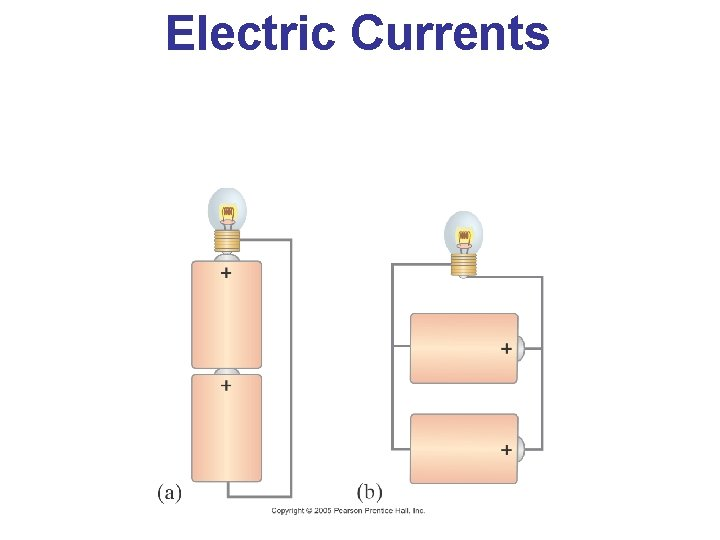Electric Currents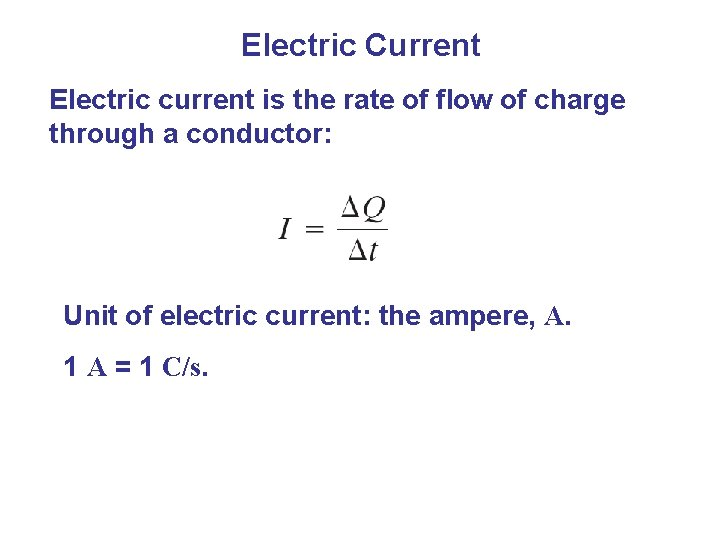Electric Current Electric current is the rate of flow of charge through a conductor: Unit of electric current: the ampere, A. 1 A = 1 C/s.Electric Current A complete circuit is one where current can flow all the way around. Note that the schematic drawing doesn’t look much like the physical circuit!Electric Current In order for current to flow, there must be a path from one battery terminal, through the circuit, and back to the other battery terminal. Only one of these circuits will work: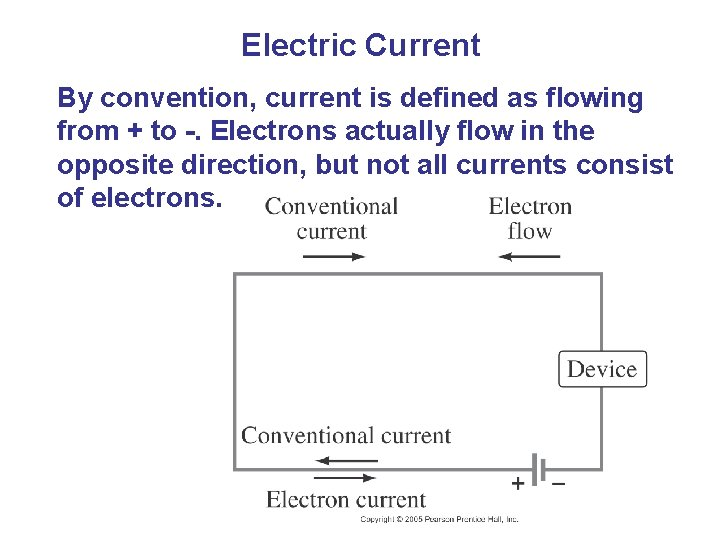Electric Current By convention, current is defined as flowing from + to -. Electrons actually flow in the opposite direction, but not all currents consist of electrons.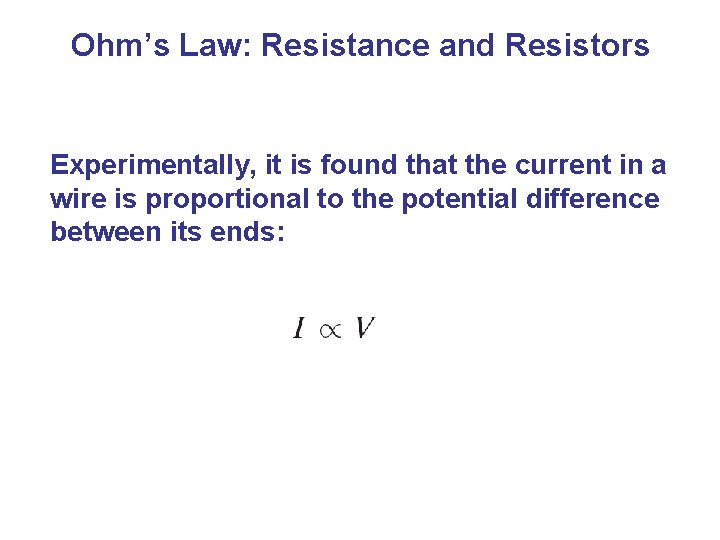Ohm’s Law: Resistance and Resistors Experimentally, it is found that the current in a wire is proportional to the potential difference between its ends: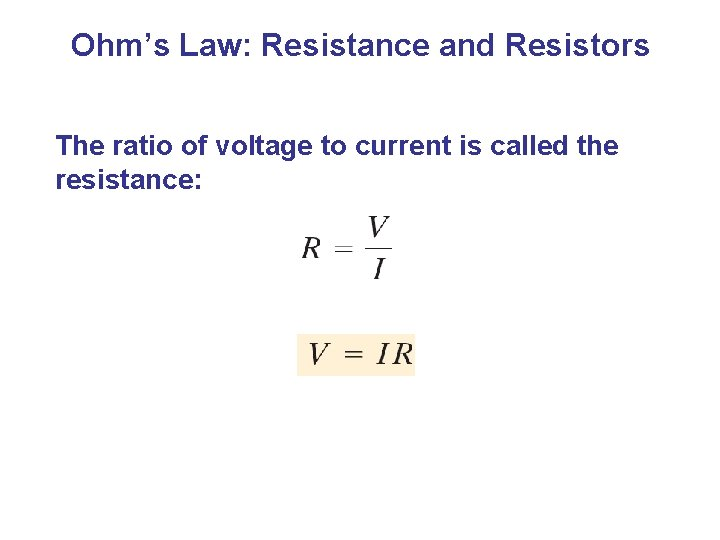Ohm’s Law: Resistance and Resistors The ratio of voltage to current is called the resistance: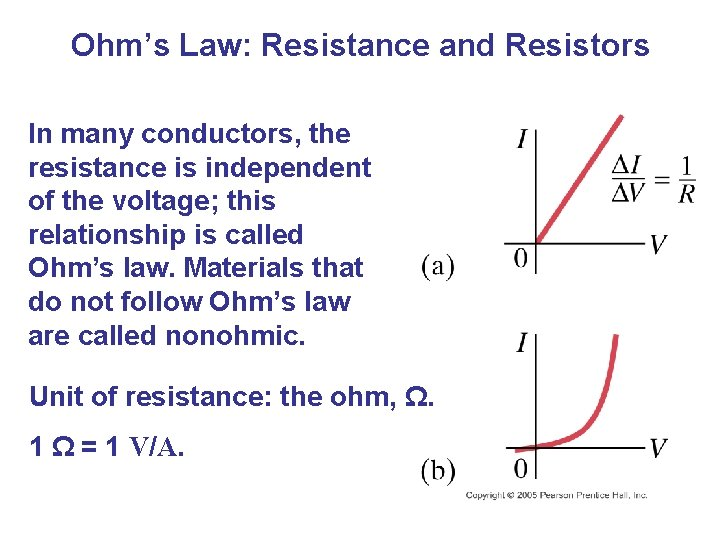Ohm’s Law: Resistance and Resistors In many conductors, the resistance is independent of the voltage; this relationship is called Ohm’s law. Materials that do not follow Ohm’s law are called nonohmic. Unit of resistance: the ohm, Ω. 1 Ω = 1 V/A.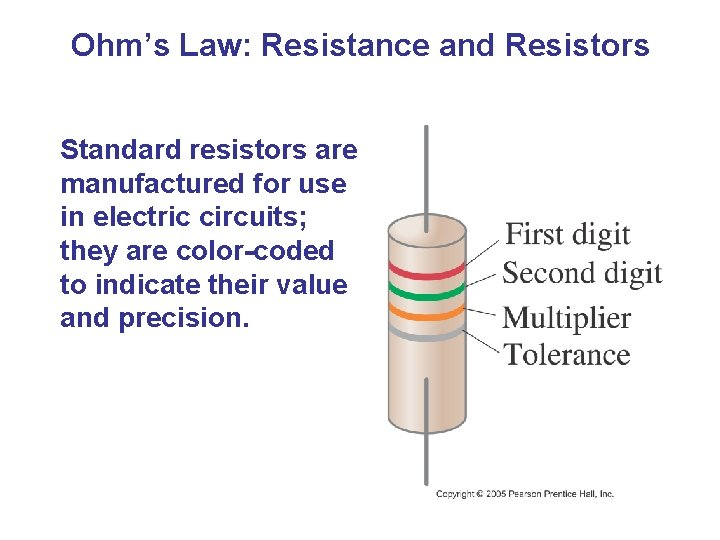Ohm’s Law: Resistance and Resistors Standard resistors are manufactured for use in electric circuits; they are color-coded to indicate their value and precision.Ohm’s Law: Resistance and Resistors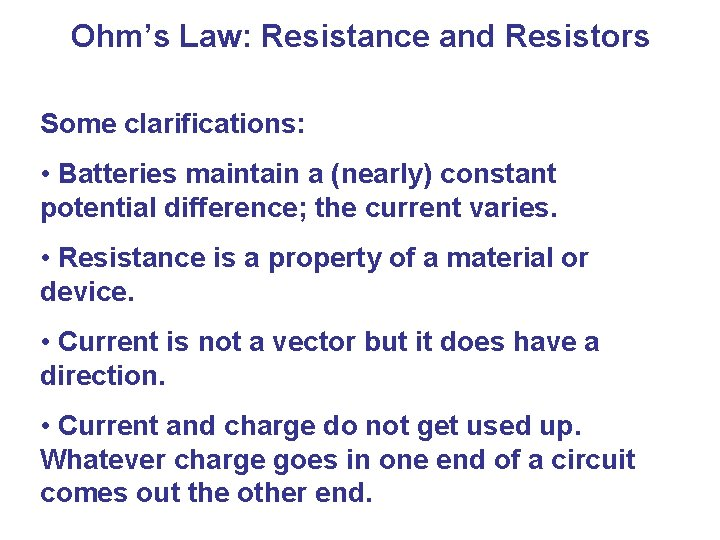Ohm’s Law: Resistance and Resistors Some clarifications: • Batteries maintain a (nearly) constant potential difference; the current varies. • Resistance is a property of a material or device. • Current is not a vector but it does have a direction. • Current and charge do not get used up. Whatever charge goes in one end of a circuit comes out the other end.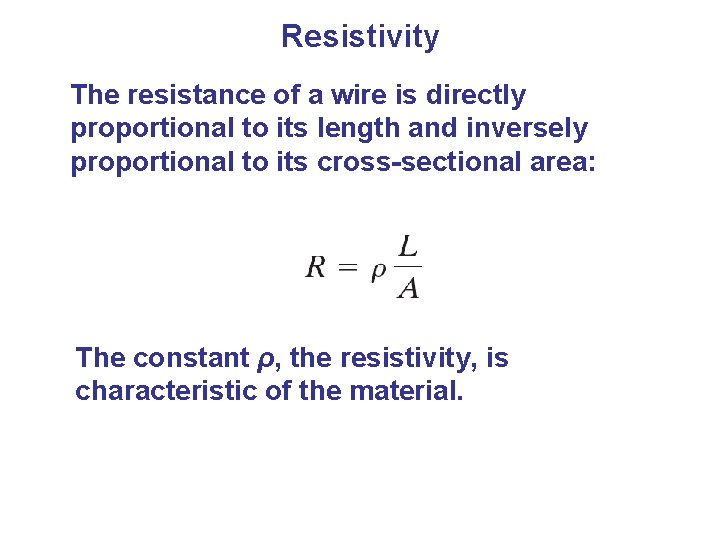Resistivity The resistance of a wire is directly proportional to its length and inversely proportional to its cross-sectional area: The constant ρ, the resistivity, is characteristic of the material.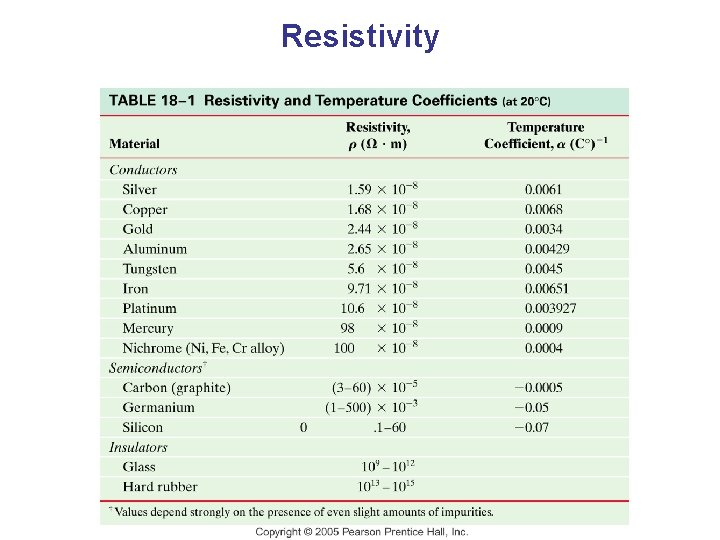ResistivityElectric Power, as in kinematics, is the energy transformed by a device per unit time:Electric Power The unit of power is the watt, W. For ohmic devices, we can make the substitutions: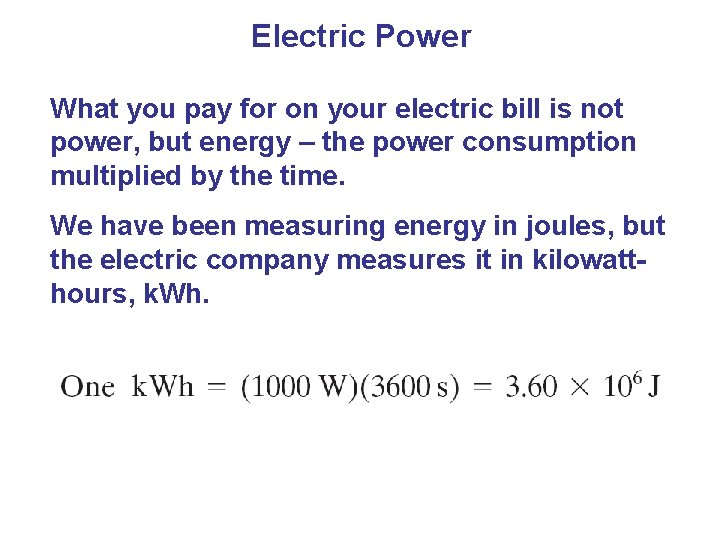Electric Power What you pay for on your electric bill is not power, but energy – the power consumption multiplied by the time. We have been measuring energy in joules, but the electric company measures it in kilowatthours, k. Wh.Power in Household Circuits The wires used in homes to carry electricity have very low resistance. However, if the current is high enough, the power will increase and the wires can become hot enough to start a fire. To avoid this, we use fuses or circuit breakers, which disconnect when the current goes above a predetermined value.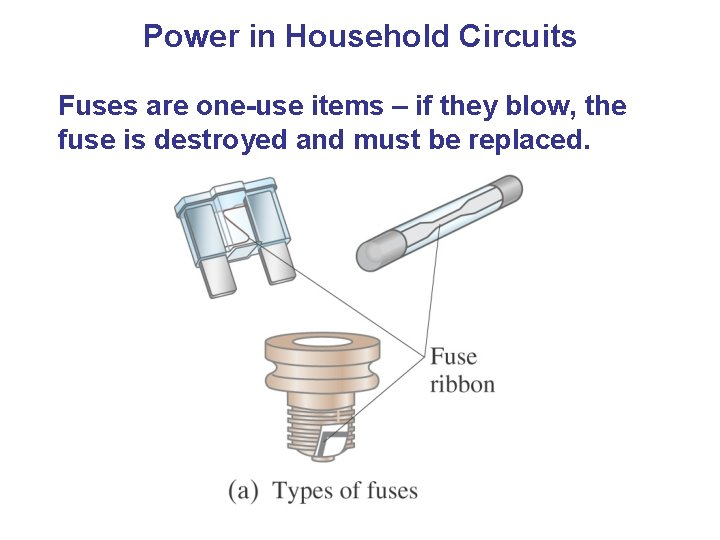Power in Household Circuits Fuses are one-use items – if they blow, the fuse is destroyed and must be replaced.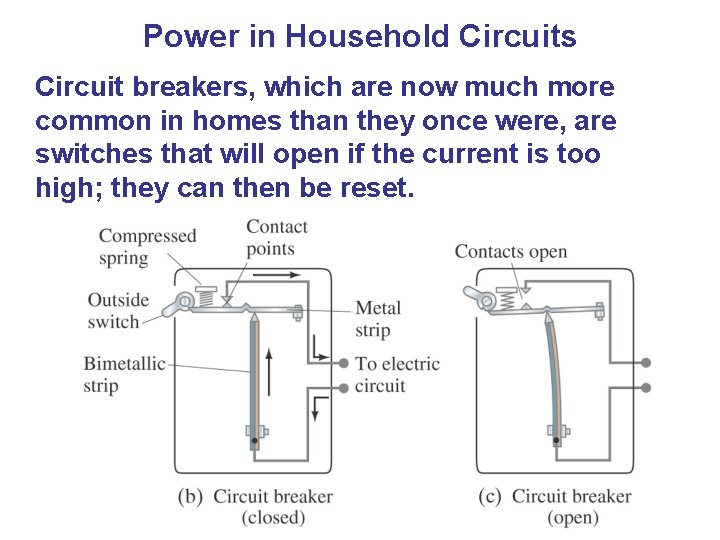Power in Household Circuits Circuit breakers, which are now much more common in homes than they once were, are switches that will open if the current is too high; they can then be reset.Microscopic View of Electric Current Electrons in a conductor have large, random speeds just due to their temperature. When a potential difference is applied, the electrons also acquire an average drift velocity, which is generally considerably smaller than thermal velocity.Superconductivity In general, resistivity decreases as temperature decreases. Some materials, however, have resistivity that falls abruptly to zero at a very low temperature, called the critical temperature, TC.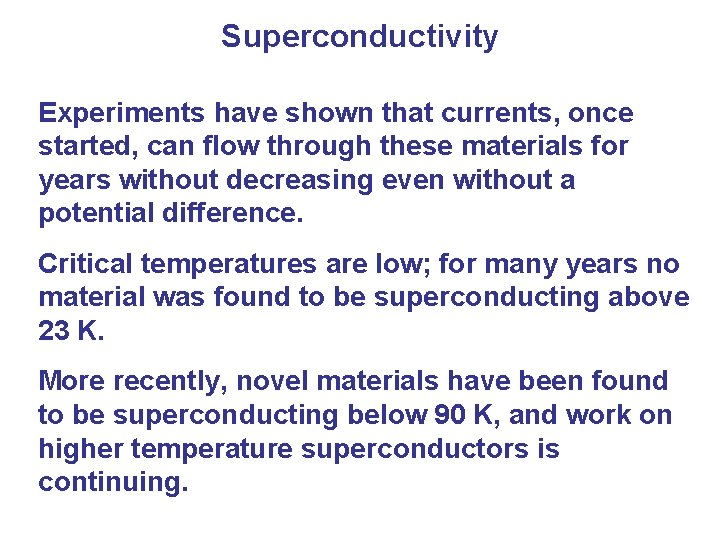Superconductivity Experiments have shown that currents, once started, can flow through these materials for years without decreasing even without a potential difference. Critical temperatures are low; for many years no material was found to be superconducting above 23 K. More recently, novel materials have been found to be superconducting below 90 K, and work on higher temperature superconductors is continuing.